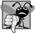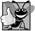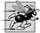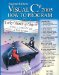# Examples Using the for Statement

The following examples show techniques for varying the control variable in a for statement. In each case, we write the appropriate for header. Note the change in the relational operator for loops that decrement the control variable.

1. Vary the control variable from 1 to 100 in increments of 1.

```for ( int i = 1; i <= 100; i++ )
```
2. Vary the control variable from 100 to 1 in decrements of 1.

```for ( int i = 100; i >= 1; i-- )
```
3. Vary the control variable from 7 to 77 in increments of 7.

```for ( int i = 7; i <= 77; i += 7 )
```
4. Vary the control variable from 20 to 2 in decrements of 2.

```for ( int i = 20; i >= 2; i -= 2 )
```
5. Vary the control variable over the following sequence of values: 2, 5, 8, 11, 14, 17, 20.

```for ( int i = 2; i <= 20; i += 3 )
```
6. Vary the control variable over the following sequence of values: 99, 88, 77, 66, 55, 44, 33, 22, 11, 0.

```for ( int i = 99; i >= 0; i -= 11 )
```## Common Programming Error 6 6

Not using the proper relational operator in the loop-continuation condition of a loop that counts downward (e.g., using i <= 1 instead of i >= 1 in a loop counting down to 1) is a logic error.

Application: Summing the Even Integers from 2 to 20

We now consider two sample applications that demonstrate simple uses of for. The application in Fig. 6.5 uses a for statement to sum the even integers from 2 to 20 and store the result in an int variable called total.

Figure 6.5. Summing integers with the for statement.

``` 1 // Fig. 6.5: Sum.cs
2 // Summing integers with the for statement.
3 using System;
4
5 public class Sum
6 {
7 public static void Main( string[] args )
8 {
9 int total = 0; // initialize total
10
11 // total even integers from 2 through 20
12 for ( int number = 2; number <= 20; number += 2 )
13  total += number;
14
15 Console.WriteLine( "Sum is {0}", total ); // display results
16 } // end Main
17 } // end class Sum
```

 ```Sum is 110 ```

The initialization and increment expressions can be comma-separated lists of expressions that enable you to use multiple initialization expressions or multiple increment expressions. For example, the body of the for statement in lines 1213 of Fig. 6.5 could be merged into the increment portion of the for header by using a comma as follows:

```for ( int number = 2; number <= 20; total += number, number += 2 )
; // empty statement
```## Good Programming Practice 6 4

Limit the size of control statement headers to a single line if possible.## Good Programming Practice 6 5

Place only expressions involving the control variables in the initialization and increment sections of a for statement. Manipulations of other variables should appear either before the loop (if they execute only once, like initialization statements) or in the body of the loop (if they execute once per iteration of the loop, like increment or decrement statements).

Application: Compound Interest Calculations

The next application uses the for statement to compute compound interest. Consider the following problem:

A person invests \$1,000 in a savings account yielding 5% interest, compounded yearly. Assuming that all the interest is left on deposit, calculate and print the amount of money in the account at the end of each year for 10 years. Use the following formula to determine the amounts:

a = p (1 + r)n

where

p is the original amount invested (i.e., the principal)

r is the annual interest rate (e.g., use 0.05 for 5%)

n is the number of years

a is the amount on deposit at the end of the nth year.

This problem involves a loop that performs the indicated calculation for each of the 10 years the money remains on deposit. The solution is the application shown in Fig. 6.6. Lines 911 in method Main declare decimal variables amount and principal, and double variable rate. Lines 1011 also initialize principal to 1000 (i.e., \$1000.00) and rate to 0.05. C# treats real number constants like 0.05 as type double. Similarly, C# treats whole number constants like 7 and 1000 as type int. When principal is initialized to 1000, the value 1000 of type int is promoted to a decimal type implicitlyno cast is required.

Figure 6.6. Compound-interest calculations with for.

(This item is displayed on page 233 in the print version)

``` 1 // Fig. 6.6: Interest.cs
2 // Compound-interest calculations with for.
3 using System;
4
5 public class Interest
6 {
7 public static void Main( string[] args )
8 {
9 decimal amount; // amount on deposit at end of each year
10 decimal principal = 1000; // initial amount before interest
11 double rate = 0.05; // interest rate
12
14 Console.WriteLine( "{0}{1,20}", "Year", "Amount on deposit" );
15
16 // calculate amount on deposit for each of ten years
17 for ( int year = 1; year <= 10; year++ )
18 {
19  // calculate new amount for specified year
20  amount = principal *
21  ( ( decimal ) Math.Pow( 1.0 + rate, year ) );
22
23  // display the year and the amount
24  Console.WriteLine( "{0,4}{1,20:C}", year, amount );
25 } // end for
26 } // end Main
27 } // end class Interest
```

 ```Year Amount on deposit 1 \$1,050.00 2 \$1,102.50 3 \$1,157.63 4 \$1,215.51 5 \$1,276.28 6 \$1,340.10 7 \$1,407.10 8 \$1,477.46 9 \$1,551.33 10 \$1,628.89 ```

Line 14 outputs the headers for the application's two columns of output. The first column displays the year, and the second column displays the amount on deposit at the end of that year. Note that we use the format item {1,20} to output the string "Amount on deposit". The integer 20 after the comma indicates that the value output should be displayed with a field width of 20that is, WriteLine displays the value with at least 20 character positions. If the value to be output is less than 20 character positions wide (17 characters in this example), the value is right justified in the field by default (in this case the value is preceded by three blanks). If the year value to be output were more than four character positions wide, the field width would be extended to the right to accommodate the entire valuethis would push the amount field to the right, upsetting the neat columns of our tabular output. To indicate that output should be left justified, simply use a negative field width.

The for statement (lines 1725) executes its body 10 times, varying control variable year from 1 to 10 in increments of 1. This loop terminates when control variable year becomes 11. (Note that year represents n in the problem statement.)

Classes provide methods that perform common tasks on objects. In fact, most methods must be called on a specific object. For example, to output a greeting in Fig. 4.2, we called method DisplayMessage on the myGradeBook object. Many classes also provide methods that perform common tasks and do not need to be called on objects. Such methods are called static methods. For example, C# does not include an exponentiation operator, so the designers of C#'s Math class defined static method Pow for raising a value to a power. You can call a static method by specifying the class name followed by the dot operator (.) and the method name, as in

```ClassName.methodName( arguments )
```

Note that Console methods Write and WriteLine are static methods. In Chapter 7, you will learn how to implement static methods in your own classes.

We use static method Pow of class Math to perform the compound interest calculation in Fig. 6.6. Math.Pow(x, y) calculates the value of x raised to the yth power. The method receives two double arguments and returns a double value. Lines 2021 perform the calculation a = p (1 + r )n, where a is the amount, p is the principal, r is the rate and n is the year. Notice that in this calculation, we need to multiply a decimal value (principal) by a double value (the return value of Math.Pow). C# will not implicitly convert double to a decimal type, or vice versa, because of the possible loss of information in either conversion, so line 21 contains a (decimal) cast operator that explicitly converts the double return value of Math.Pow to a decimal.

After each calculation, line 24 outputs the year and the amount on deposit at the end of that year. The year is output in a field width of four characters (as specified by {0,4}). The amount is output as a currency value with the format item {1,20:C}. The number 20 in the format item indicates that the value should be output right justified with a field width of 20 characters. The format specifier C specifies that the number should be formatted as currency.

Notice that we declared the variables amount and principal to be of type decimal rather than double. Recall that we introduced type decimal for monetary calculations in Section 4.10. We also use decimal in Fig. 6.6 for this purpose. You may be curious as to why we do this. We are dealing with fractional parts of dollars and thus need a type that allows decimal points in its values. Unfortunately, floating-point numbers of type double (or float) can cause trouble in monetary calculations. Two double dollar amounts stored in the machine could be 14.234 (which would normally be rounded to 14.23 for display purposes) and 18.673 (which would normally be rounded to 18.67 for display purposes). When these amounts are added, they produce the internal sum 32.907, which would normally be rounded to 32.91 for display purposes. Thus, your output could appear as

``` 14.23
+ 18.67
-------
32.91
```

but a person adding the individual numbers as displayed would expect the sum to be 32.90. You have been warned! For people who work with programming languages that do not support a type for precise monetary calculations, Exercise 6.18 explores the use of integers to perform such calculations.## Good Programming Practice 6 6

Do not use variables of type double (or float) to perform precise monetary calculations; use type decimal instead. The imprecision of floating-point numbers can cause errors that will result in incorrect monetary values.

Note that the body of the for statement contains the calculation 1.0 + rate, which appears as an argument to the Math.Pow method. In fact, this calculation produces the same result each time through the loop, so repeating the calculation in every iteration of the loop is wasteful.## Performance Tip 6 2

In loops, avoid calculations for which the result never changessuch calculations should typically be placed before the loop. [Note: Optimizing compilers will typically place such calculations outside loops in the compiled code.]Visual C# 2005 How to Program (2nd Edition)
ISBN: 0131525239
EAN: 2147483647
Year: 2004
Pages: 600